# 3. Answer to the followings (30 points): (a) Find orthonormal vectors u2 93 such that q1,...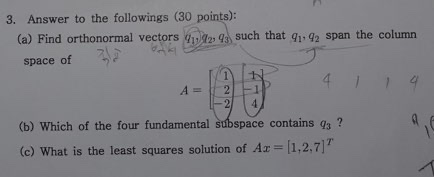3. Answer to the followings (30 points): (a) Find orthonormal vectors u2 93 such that q1, 92 span the column 3/2 space of 2. -2 4. (b) Which of the four fundamental subspace contains 93 (c) What is the least squares solution of Ar= 1,2,7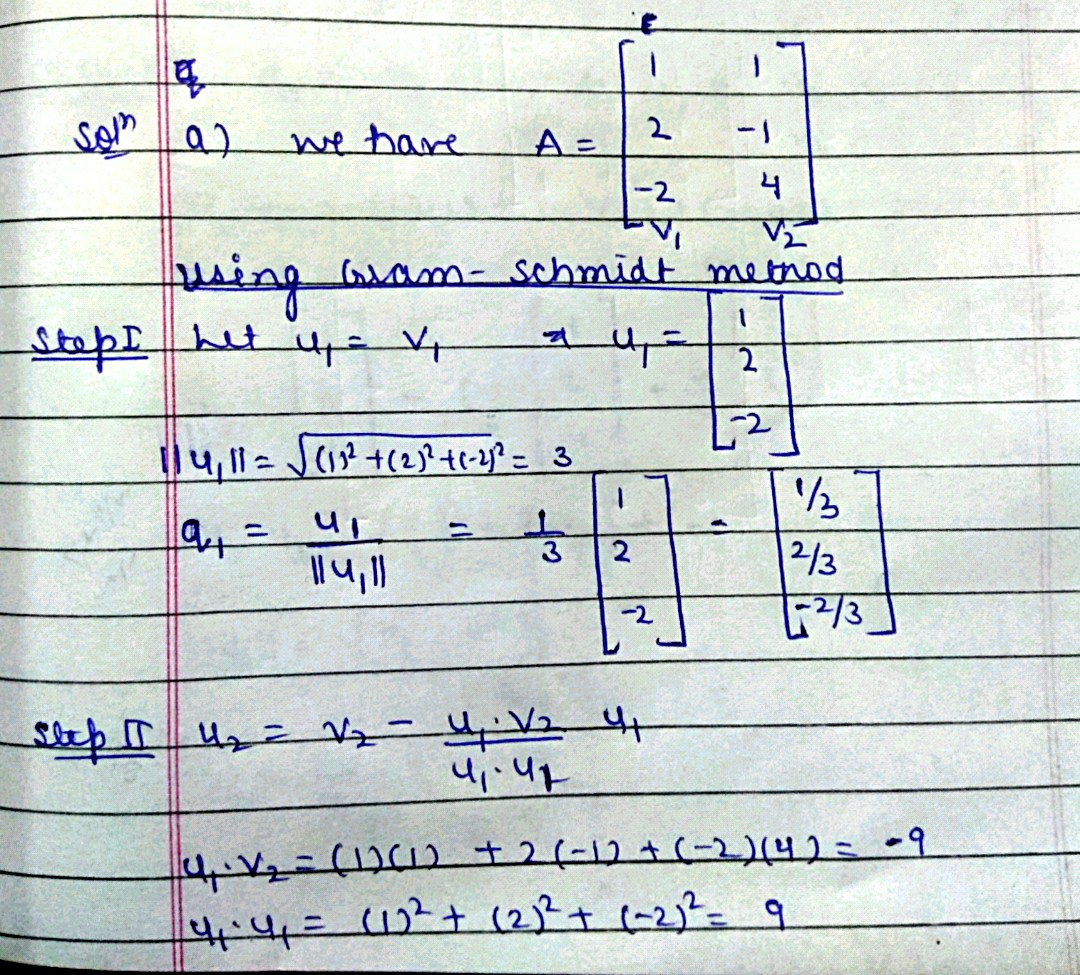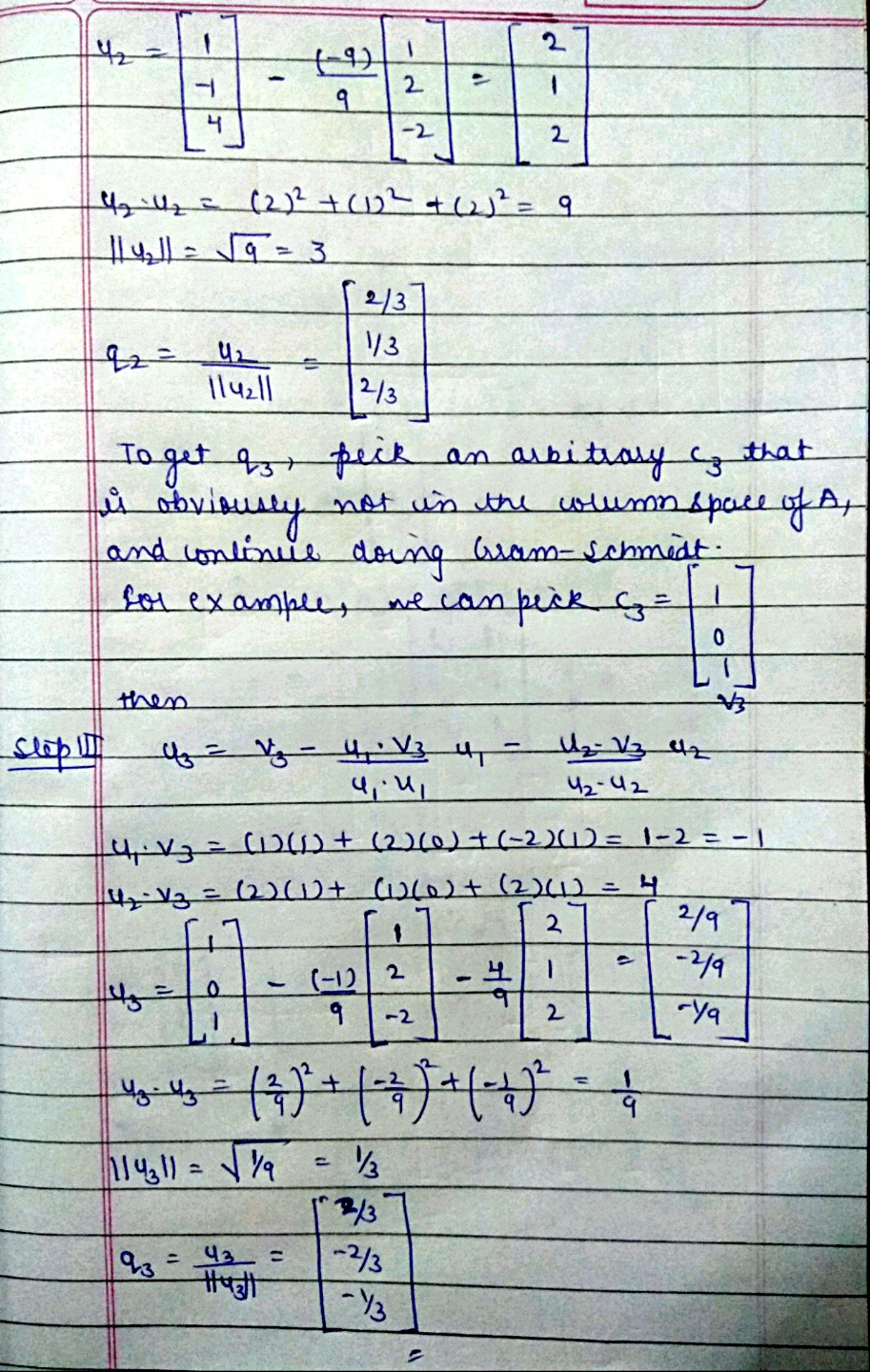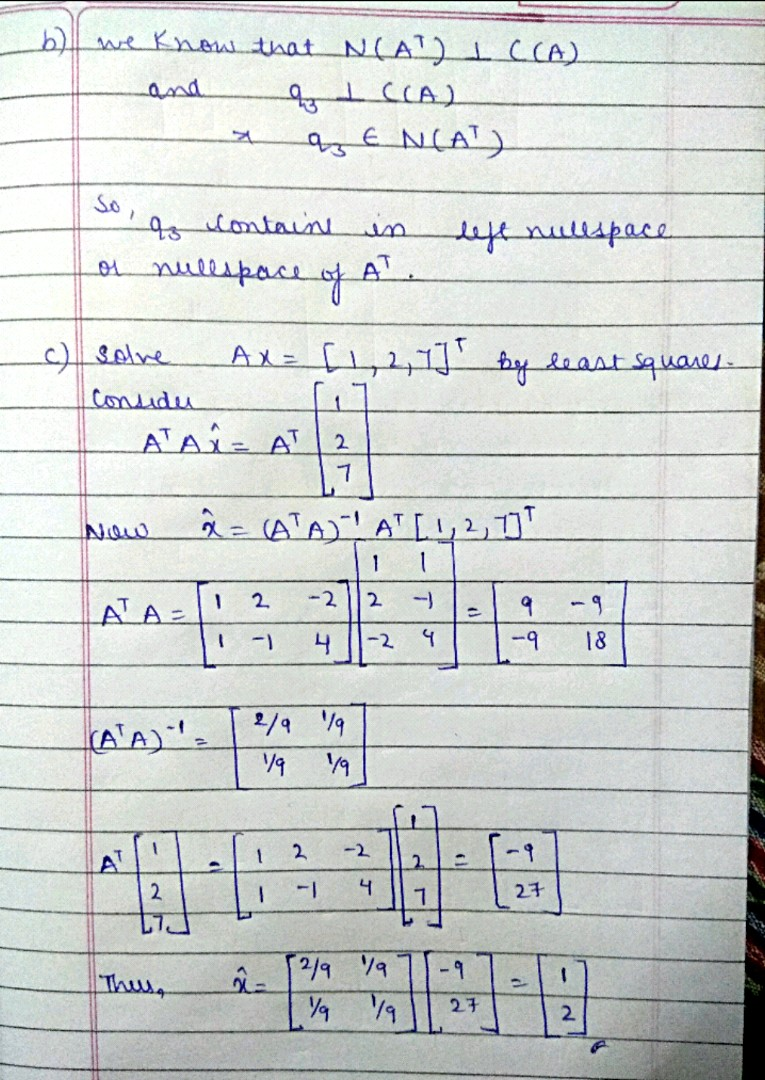##### Add Answer to: 3. Answer to the followings (30 points): (a) Find orthonormal vectors u2 93 such that q1,...
Similar Homework Help Questions
• ### How can I get the (a) 3*2 matrix A? x 7. [30pts] Let V be the subspace of R consisting of vectors satisfying x- y+z = 0...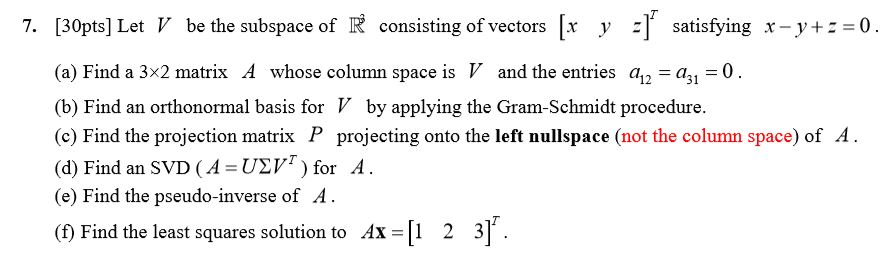How can I get the (a) 3*2 matrix A? x 7. [30pts] Let V be the subspace of R consisting of vectors satisfying x- y+z = 0 y (a) Find a 3x2 matrix A whose column space is V and the entries a a1 0 = (b) Find an orthonormal basis for V by applying the Gram-Schmidt procedure (c) Find the projection matrix P projecting onto the left nullspace (not the column space) of A (d) Find an SVD (A...

• ### Please attempt both questions. 5. Find an orthonormal basis for the plane viewed as a subspace...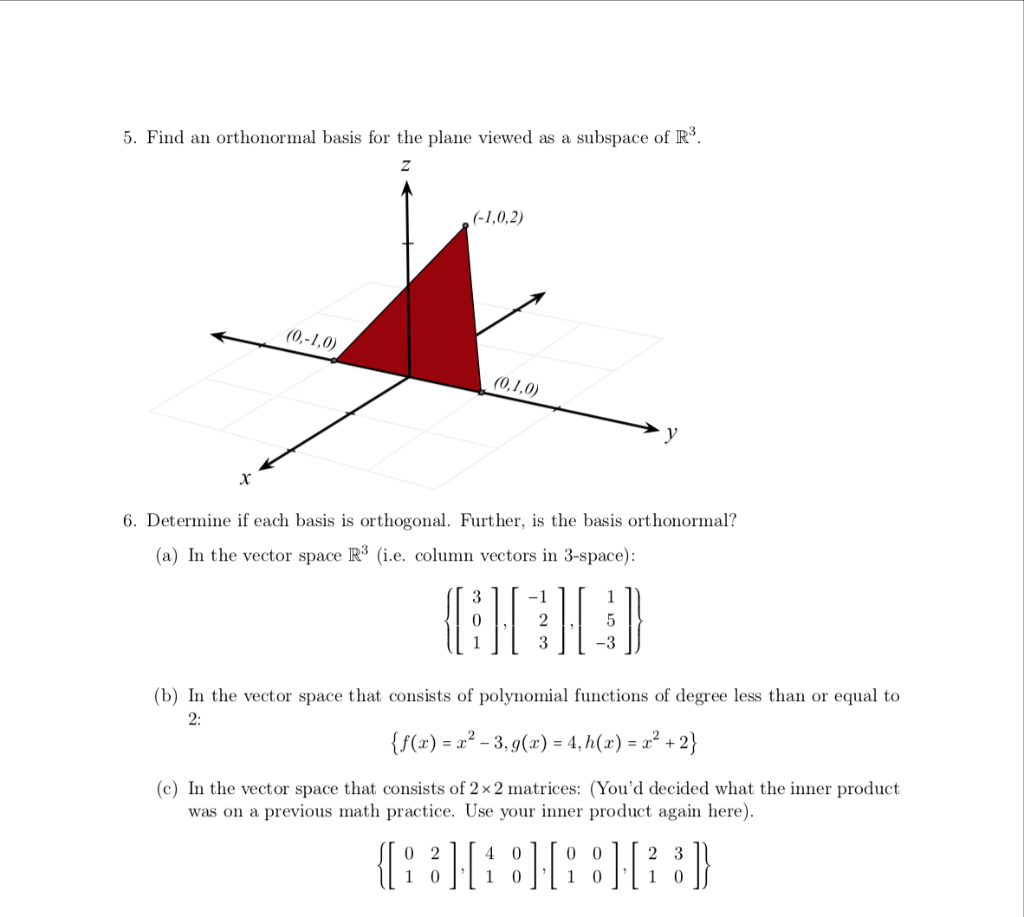Please attempt both questions. 5. Find an orthonormal basis for the plane viewed as a subspace of R3. Z (-1,0,2) (0,-1,0) (0,1,0) X 6. Determine if each basis is orthogonal. Further, is the basis orthonormal? (a) In the vector space R3 (i.e. column vectors in 3-space): 1 2 5 -3 (b) In the vector space that consists of polynomial functions of degree less than or equal to 2: {f(x) = 22 - 3, 9() = 4, h(x) = 2² +2}...

• ### ote: The norm of is denoted by |vand is calculated N a vector u Consider a subspace W of R4, W span(1, v2, v3, v4)). Where 0 из- 1. Find an orthonormal basis Qw of W and find the dimension of W 2. Fi...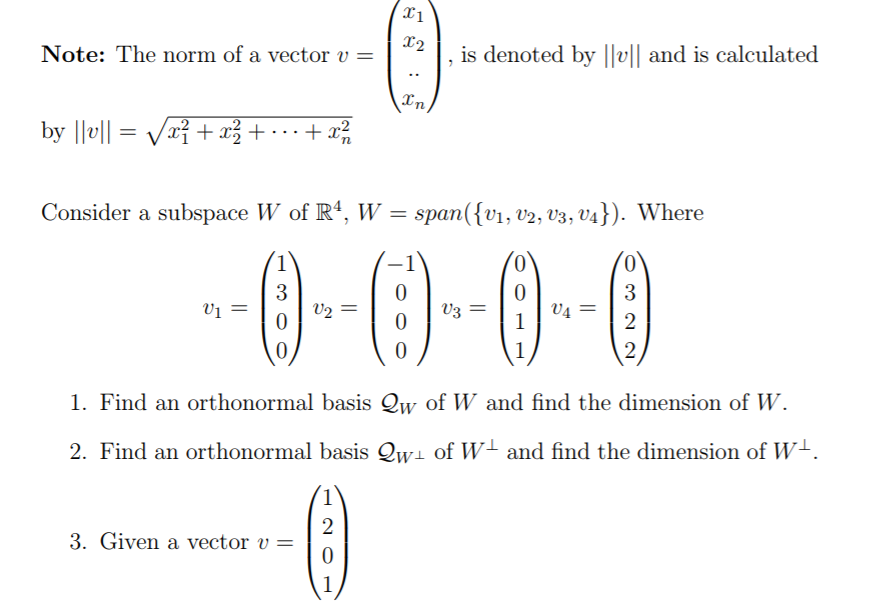ote: The norm of is denoted by |vand is calculated N a vector u Consider a subspace W of R4, W span(1, v2, v3, v4)). Where 0 из- 1. Find an orthonormal basis Qw of W and find the dimension of W 2. Find an orthonormal basis QWL of WL and find the dimension of WL 3. GIven a vector u- . find the Qw coordinate of Projw(v) . find the Qwa coordinate of Projwi (v) » find the coordinate...

• ### =E- 3 1 Q1: Consider the complex vectors: ū = 21, ý = 1 - 2...=E- 3 1 Q1: Consider the complex vectors: ū = 21, ý = 1 - 2 -5 a) Evaluate <ü, lv > where 1 = 2 - i. b) Find the distance between ū and . c) Decide whether vectors ū and v are orthonormal. d) Describe the span of the vectors ū and v.

• ### 22. (a) Find two vectors that span the null space of A 3 -1 2 -4 (b) Use the result of part (a) to find the matrix that projects vectors onto the null space of A. (c) Find two orthogonal vectors...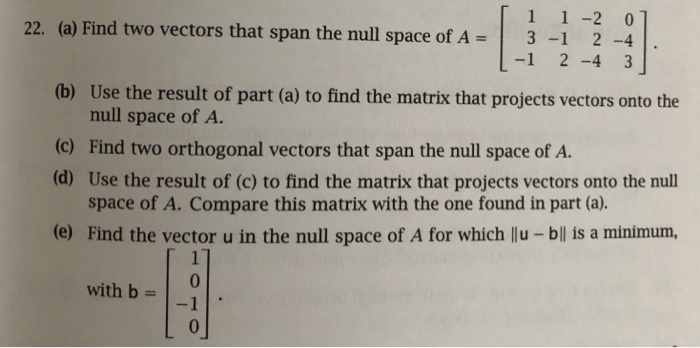22. (a) Find two vectors that span the null space of A 3 -1 2 -4 (b) Use the result of part (a) to find the matrix that projects vectors onto the null space of A. (c) Find two orthogonal vectors that span the null space of A. (d) Use the result of (c) to find the matrix that projects vectors onto the nul space of A. Compare this matrix with the one found in part (a). (e) Find the...

• ### [21 points, as followa [2 point] 12 points] (4 pointa) 10. Find a basis for the...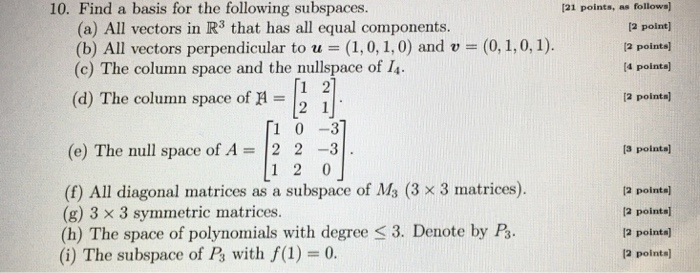[21 points, as followa [2 point] 12 points] (4 pointa) 10. Find a basis for the following subspaces. (a) All vectors in R3 that has all equal components. (b) All vectors perpendicular to u (1, 0, 1,0) and v (0, 1,0,1). (c) The column space and the nullspace of I4 2 (d) The column space of 2 points) 1 0-3 (e) The null space of A2 23 [3 pointa) 1 2 0 (f) All diagonal matrices as a subspace of...

• ### LINEAR ALGEBRA MATLAB HOMEWORK HELP!!! THANKS   5. Let W be the subspace of R5 given by 3 24 216 -15 3 13 (a) Enter the four vectors into MATLAB as vi, v2, v3 and v4 respectively b) Let A- [v1 v2 v3...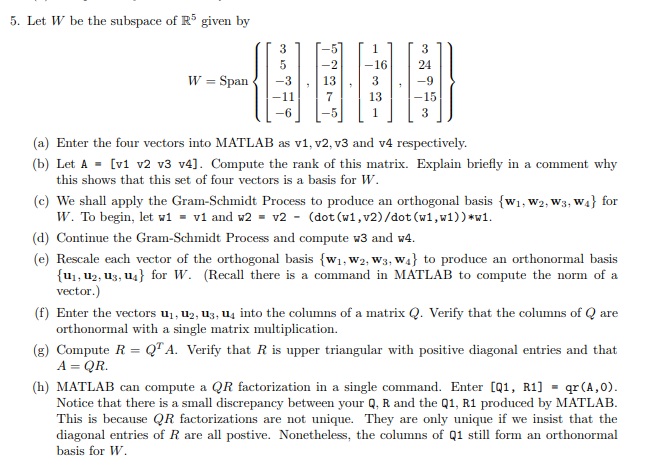LINEAR ALGEBRA MATLAB HOMEWORK HELP!!! THANKS   5. Let W be the subspace of R5 given by 3 24 216 -15 3 13 (a) Enter the four vectors into MATLAB as vi, v2, v3 and v4 respectively b) Let A- [v1 v2 v3 v4]. Compute the rank of this matrix. Explain briefly in a comment why (c) We shall apply the Gram-Schmidt Process to produce an orthogonal basis [wi, W2, ws, wał for (d) Continue the Gram-Schmidt Process and compute w3...

• ### linear algebra question 0. Given 1 3-5 1 1 -2 1-3 1 and b If the Gram-Schmidt process is applied to determine an orthonormal basis for R(A), and a QR factoriza- tion of A then, after the first two or...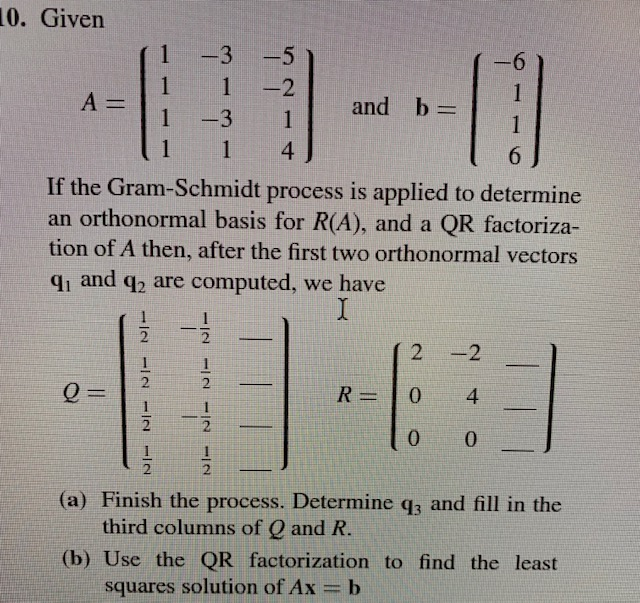linear algebra question 0. Given 1 3-5 1 1 -2 1-3 1 and b If the Gram-Schmidt process is applied to determine an orthonormal basis for R(A), and a QR factoriza- tion of A then, after the first two orthonormal vectors qi and q are computed, we have 2 -2 2 2 2 2 2 (a) Finish the process. Determine q3 and fill in the third columns of Q and R (b) Use the QR factorization to find the least...

• ### I need help with parts c and d of this question. Some concept clarification would be great. 3. Consider the following matrix A= 3 6 (a) Compute AAT and its eigenvalues and unit eigenvectors. (b) Find...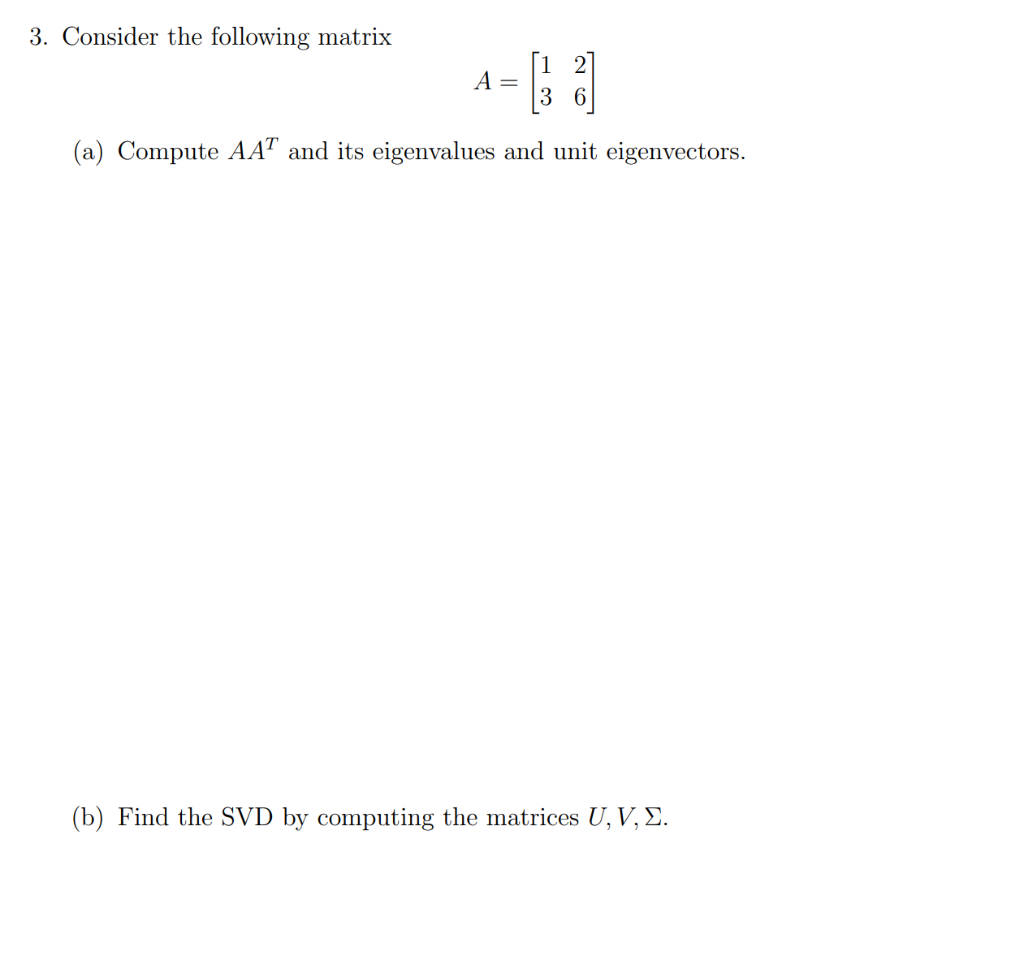I need help with parts c and d of this question. Some concept clarification would be great. 3. Consider the following matrix A= 3 6 (a) Compute AAT and its eigenvalues and unit eigenvectors. (b) Find the SVD by computing the matrices U, V, Σ (c) From the u's and v's in (b), write down orthonormal bases for all four fundamental subspaces (i.e., row space, column space, null space, left null space) of the matrix A. (d) Compute the pseudoinverse...

• ### Find the best approximation to z by vectors of the form C7 V + c2V2. 3...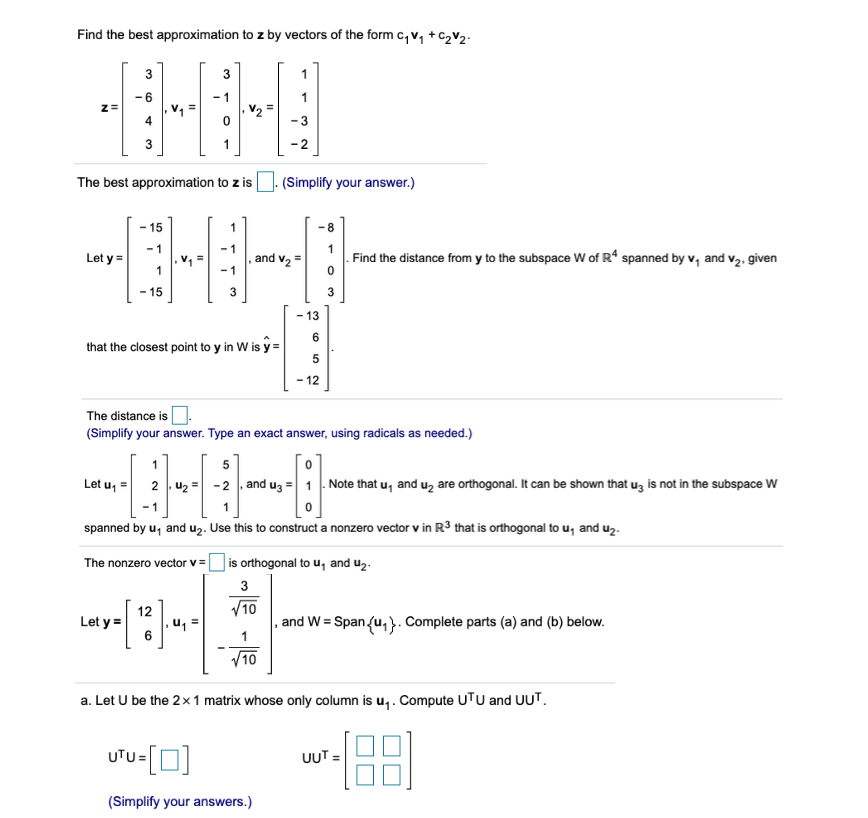Find the best approximation to z by vectors of the form C7 V + c2V2. 3 1 3 -1 -6 1 z = V2 4 0 -3 3 1 The best approximation to z is . (Simplify your answer.) - 15 - 8 8 - 1 Let y = , and v2 Find the distance from y to the subspace W of R* spanned by V, and vą, given 1 0 1 - 15 3 3 - 13 09 that...

Free Homework App